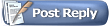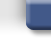Project64 ForumsBlog Space :D Sine of One Degree
 FAQ Members List Calendar Search Today's Posts Mark Forums Read#31HatCatAlpha Tester Project Supporter Senior Member Join Date: Feb 2007 Location: In my hat. Posts: 16,236Frekkin' sweet. Finally I've got (a * a).
Code:
```var srt3 = Math.sqrt(3);
var srt15 = Math.sqrt(15);
var disc = Math.sqrt(10 - 2 * Math.sqrt(5));
number = 2048 - 256 * srt3 - 256 * srt15 - 256 * disc;```
That returns +11.2191582857763.

My ActionScript code for just (a) from page 1 of this thread,
Code:
```var srt2 = Math.sqrt(2);
var srt3 = Math.sqrt(3);
var srt5 = Math.sqrt(5);
var srt6 = Math.sqrt(6);
var disc = Math.sqrt(25 + (11 * srt5));
number = (4 * disc * (srt3 - 1) * (3 - srt5)) + (4 * (srt6 + srt2) * (1 - srt5));```
Returned -3.34950119954841.

Square that in Windows Calculator, it returns about +11.219. Finally, after so much hazard-prone writing in pitch-black ink.Well it's gel pen or something I think, but who cares I just cross it out with a simple strikeout rather than smear it with pencil scribbles like people usually do.

combo breaker post for status summary heh...
#32HatCatAlpha Tester Project Supporter Senior Member Join Date: Feb 2007 Location: In my hat. Posts: 16,236Basically everything about the setup expression so far.Except for neatness on the right-hand sides of the statements I don't directly define the square of a or other expressions, since the factorization is more clean.

Compared to the amount of time and pages it took to do that, finding 2ab and the square of b should be probably about 10 percent the remaining work.
#33HatCatAlpha Tester Project Supporter Senior Member Join Date: Feb 2007 Location: In my hat. Posts: 16,236Combining like terms from within solutions already found is actually taking a while though, so I'd best backup the decimal constant equivalencies of both squares.

Code:
```(a * a) ~=   +11.2191582857763
(b * b) ~= -4084.7808417142200
//     s = a * a + b * b
s       ~= -4073.5616834284437```
This helps speed up debugging any persistent hazards in the writing.
#34NatchProject Supporter Senior Member Join Date: Jan 2011 Location: Nowhere near you. Posts: 5,078Oh, the sine of one degree. GotchaNo I really had no idea what you were trying to do. Like I said, maths....
__________________#35HatCatAlpha Tester Project Supporter Senior Member Join Date: Feb 2007 Location: In my hat. Posts: 16,236You can't define the exact value of pi using a finite expression of radicals of integers, but you can define the sine, cosine, tangent, co-secant, secant, and cotangent of an angle's measure in that way. Again, it's almost useless in software engineering because of the way floating-point numbers are found or stored from reading/writing bits, but it's the only way to have the "source code" behind some messy floating-point decimal you use a calculator to get.

The trigonometric functions obviously work with triangle geometry, planar in the second dimension, so finite expressions of square roots can be used to define.

The pi constant is approximated using infinite sequences or series. It's kind of like a regular (all angles congruent, all sides congruent) polygon with n sides: As n increases, the polygon closer resembles to a circle (which could be thought of as a regular polygon with infinite sides), but infinity is not a real number. Then there is no finite way to define the ratio of a circle's circumference to its diameter.

Last edited by HatCat; 9th July 2011 at 11:06 PM.
#36NatchProject Supporter Senior Member Join Date: Jan 2011 Location: Nowhere near you. Posts: 5,078Do you know what he's talking about, Squall?

"Yes.
wait... lol wut"
__________________#37HatCatAlpha Tester Project Supporter Senior Member Join Date: Feb 2007 Location: In my hat. Posts: 16,236In other words, RTFM.
#38HatCatAlpha Tester Project Supporter Senior Member Join Date: Feb 2007 Location: In my hat. Posts: 16,236Using a rewritten expression for the square of b, finding the sum of the squares of a and b and simplifying it was easy.

That expression can be factored as:
(a^2 + b^2):512 = [sqrt(2)][2 - sqrt(5)][sqrt(25 + 11(sqrt(5)))] - [sqrt(3)][1 + sqrt(5)]

The problem is the length of writing in finding the product of a and b.Finishing writing the steps out for that will take a while.
#39HatCatAlpha Tester Project Supporter Senior Member Join Date: Feb 2007 Location: In my hat. Posts: 16,236Long time, no update. This is because I've sidetracked my mind onto other things.Here is some more summarizing info on the components to the sine of one degree.So, again, I've had the sum of the squares of a and b. I also have most of the combined (not factorized) expression equivalent to one-sixty-fourth the product of a and b written across a sheet of paper, but that would have flooded the GIF picture.

How important is it that I define a * b / 64 without using factorization to shorten the amount of writing? As it turns out, probably not that important, because while the end result will still be in terms of the imaginary unit i = Math.sqrt(-1), the binomial that is the sum of a and b in the denominator of the ratio must be multiplied by its conjugate, along with the numerator of the ratio, to rationalize the denominator of the resulting expression.

Also, a final significant question, is how do I know that the writer of the process guide for deriving the sine of one degree is right in saying that the expression is ultimately a real number of the form a + 0i and that all instances of i are "cancelled out" in the final expression? Answer being, there's only one way to find out.I can't prove it's impossible just yet, so I'm just presuming I haven't been misled for now.Last edited by HatCat; 22nd July 2011 at 02:10 AM.
#40HatCatAlpha Tester Project Supporter Senior Member Join Date: Feb 2007 Location: In my hat. Posts: 16,236Just a technical update here. I haven't felt in the mood (not tired of mathjust mood issues elsewhere) to write more solutions of this, but I have confirmed two things I want to do.

One thing, is that, since b is an imaginary number in terms of the imaginary unit, the square root of negative one, the denominator of the original ratio should be multiplied by its conjugate, the expression (a minus b).

sin(+1.) = [(a + b)(a + b) - 64]:[512(a + b)]
sin(+1.) = [(a + b)(a + b)(a - b) - 64(a - b)]:[512(a + b)(a - b)]

The product of the sum of a and b and the difference of a and b, is the difference of the squares of a and b (or a^2 - b^2). This is fortunate because the square of b is a real number not in terms of the imaginary unit, so this rationalizes the denominator of the ratio to a real number while probably making the math easier on the numerator of the ratio as well.

The other thing is I might want to double-check that that is the correct way of defining the sine of one degree and that I didn't make a mistake defining sin(+1.) to that much smaller expression. Even if I did, the calculations I've done so far are very useful and reusable, but I can be grateful if I was wrong at this point.Last edited by HatCat; 22nd July 2011 at 11:11 PM. Reason: oops, fixed a hasty distributionThread ToolsShow Printable VersionEmail this Page Display ModesLinear ModeSwitch to Hybrid ModeSwitch to Threaded ModePosting Rules You may not post new threads You may not post replies You may not post attachments You may not edit your posts BB code is On Smilies are On [IMG] code is On HTML code is Off
 Forum Jump User Control Panel Private Messages Subscriptions Who's Online Search Forums Forums Home General Discussion     Site News     Open Discussion Public Version     Project 64 - v2.x - Suggestions     Project 64 - v2.x - Issues     Project64 - Android     Project 64 - v1.6

All times are GMT. The time now is 01:33 AM.Contact Us - Archive - Top